Next: Estimation of nonstationary PEFs Up: Geometric interpretation of the Previous: General properties of the

## Geometric interpretation

The operatorsandare the noise and signal resolution operators. They describe how well the predictions match the noise and signal Menke (1989). In the following equations, we consider that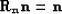(114)
and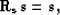(115)
meaning that each component of the data has been predicted. These equalities will help us to build a comprehensive geometric interpretation for the different operators. Based on equations () and (), we have for the data vector d the following equalities: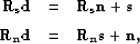(116)
and

 geom11 Figure 1 A geometric interpretation of the noise filter when n and s are not orthogonal.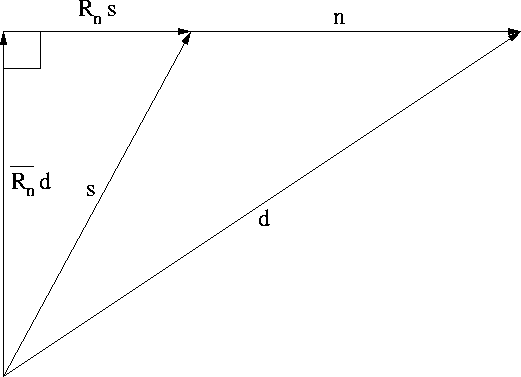geom21 Figure 2 A geometric interpretation of the noise filter when n and s are orthogonal.(117)
In the following equations, we prove that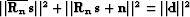: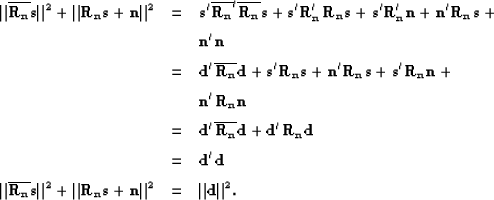(118)
Similarly, we have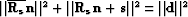. If we use equations () and (), the last two equalities can be written as follows: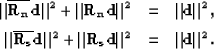(119)
Hence,,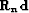and d form a right triangle with hypotenuse d and legsand, as depicted in Figure; similarly,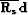,and d form a right triangle with hypotenuse d and legsand.If n and s are orthogonal, s is in the null space ofand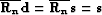(Figure). Similarly, n is in the null space ofand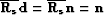.Next: Estimation of nonstationary PEFs Up: Geometric interpretation of the Previous: General properties of the
Stanford Exploration Project
5/5/2005